Do you know what JavaScript Dollar Sign is? Have you ever thought about the ground where Dollar Sign JavaScript can be implemented? If you’re curious to know about Dollar Sign variable with some methods of implementation.

In this article, we will first discuss the background of the JavaScript Dollar Sign before moving to the implementation process. We should connect the concept with some basic topics that we all know about. In this way, the topic becomes easier for us.

So, let us connect the JavaScript Dollar Sign with one of the basic concepts in computer science.

We all know that in computer science, there are many different concepts in different programming languages. Like, for taking any input from the users, in the C programming language we use the ‘scanf’ statement. In Python programming language, we use the ‘Print’ statement for that. Also, if you wish to know more about Python, you can check out our article on “How to Compare Strings In Python?”

So, in different programming languages, the same thing can be implemented using different keywords. And the JavaScript programming language is no different from that. Here, the dollar sign is used to forecast the output data.

Like, in the C programming language, we use different identifiers like %d, %s, etc. The dollar sign is used for the same purpose. The connection between the dollar sign in JavaScript & simple identifiers in C programming language must be cleared in front of you.

## What Is JavaScipt Dollar Sign? Get To Know

In one simple sentence, we can call the dollar sign in JavaScript as the identifier. As an identifier, the dollar sign in JavaScript can be utilized in any of the domains of JavaScript. It can be used as the identifier of the variable, properties, function, etc. in the JavaScript programming language.

Also, it can be used as the alphabetic character in the program. That means this element can be used inside of any variable name. And then, it will be considered as the character element. So, the use of the dollar sign in JavaScript is huge.

Every time, you will use the dollar sign for a particular use, the appearance of it will be different. That means the way to use the dollar sign for forecasting output data will be different from the identifier dollar sign. So, it will be better to focus on the use of the dollar sign for printing the output data.

In another way, the dollar sign can be used in JavaScript. In that case, the dollar sign is used as the function. And without any name of the function, such elements can execute in the program. But uses of such concepts are still under consideration.

There is no proof that the dollar sign as a function can be the same effect as in any other field in JavaScript. So, it will be better not to use such things without any prior experience.

## What Are The Prerequisites You Need To Know?

While writing codes in JavaScript, developers have a habit to use the method console for printing the output of any program. Oftentimes, such processes are followed by most developers. But, while printing the data using the dollar sign, you need to follow another way.

As a developer, your goal is to make one program more users interactive. And to make the program more users interactive, you should use the Prompt Box & Alert Box in the program. These are the concepts of the HTML programming language that we will use.

Let us have a look at the following points to get the details of the above boxes.

• Prompt Box: It is one of the browser-provided boxes, where the input from the users can be taken. And the input will be directly saved to the variable in the program. Inside the prompt box, write any statement that will be used to take the inputs. The prompt() function is used here.
• Alert Box: The alert boxes are highly used in the browser, to fetch the attention of the users. The same box can be used in the JavaScript program. Here, the box will be used to print the data. Inside the box, we will use the dollar sign & as well as some statements to provide the output. The alert() function is used in this case.

So, these are the things you should clear before moving to the implementation process. Now, if the prerequisites are cleared, then we will move to the implementation process of JavaScipt Dollar Sign.

As the dollar sign in JavaScript is used for forecasting the outputs of the program, the implementation process will be moreover same. The dollar sign can be used for any data type inside of JavaScript programming language.

1. Dollar Sign For Integer
2. Dollar Sign For String

We will know about these implementation methods one by one briefly.

### What Is The Implementation Method Of Dollar Sign For Integers?

Here, we will take two different examples. In the first case, we will use a simple implementation method. And in the second case, we will use a complex implementation method. So, let us start the implementation method.

##### Sample 1: Simple Implementation:

Here, simply we have taken one integer value in the program. Using the prompt() function, we are taking one integer value from the user. The same value is going to store one constant data inside of the program.

After taking the data, we will print the output data. Here, the input data is going to be printed without any modification. Now, the alert() function will be used to get the output of the program in the alert box.

Example:

```const i1 = prompt("Enter Any Number: "); // Declaring Variable

alert(`The Number Is: \${i1}`); // Providing Output```

Let us try to find out the output of the above code. It will help to understand the implementation process of dollar signs for simple integers.

Output: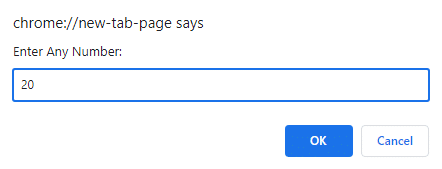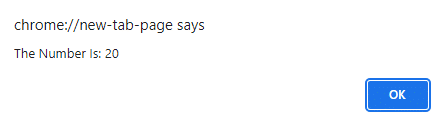##### Sample 2: Complex Implementation:

Here, we will use the knowledge of the simple implementation process & take two different integer values from the users. After that, one arithmetic operation will perform. But before that, we need to make use of parseInt().

It is the method to change the string to the integer. It is similar to typecasting which we perform in other languages. The inputs are in the string format, so it is necessary to make them the integer.

Now, like the same simple concept, we will print the output using the alert() function in the program. So, the arithmetic result will be printed now.

Example:

```const input1 = prompt("Enter Number One: "); // Declaring First Variable

const input2 = prompt("Enter Number Two: "); // Declaring Second Variable

input3=parseInt(input1)+parseInt(input2); // Type Casting To Integer & Adding Them

alert(`Result Is \${input3}`); // Providing Output```

Let us try to find out the output of the above code. It will help to understand the implementation process of dollar signs for complex integers.

Output: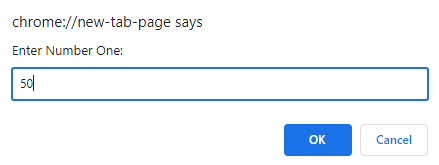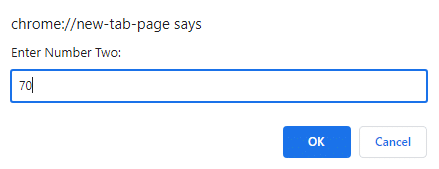### What Is The Implementation Method Of Dollar Sign For Strings?

Here again, we will take two different examples. In the first case, we will use a simple implementation method. And in the second case, we will use a complex implementation method.

##### Sample 1: Simple Implementation:

It is the complete same process that we have done for the integer case. Just here, we have used the ‘var’ keyword instead of the ‘const’ data type to accept the string.

Example:

```var c = prompt("Provide Organization Name: "); // Declaring First String

alert(`The Output Is: \${c}`); // Providing The Output```

Let us try to find out the output of the above code. It will help to understand the implementation process of dollar signs for simple strings.

Output: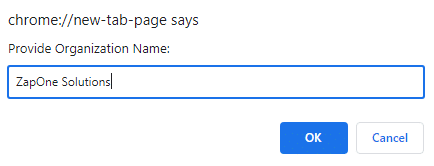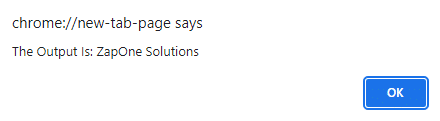##### Sample 2: Complex Implementation:

Here, we are going to follow a similar method as we have done for the simple implementation method. We will take two different string inputs from the users. And those data will be saved in different variables.

Then, using the simple concatenation concept, we are going to add those data to the program. And those data will be saved in one variable. The same variable will be used inside of the alert() function for printing purposes.

Example:

```var i = prompt("Enter First Name: "); // Declaring First String

var j = prompt("Enter Second Name: "); // Declaring Second String

k = i + ' ' + j; // Concatenation Of Strings

alert(`Full Name Is \${k}`); // Providing Output```

Let us try to find out the output of the above code. It will help to understand the implementation process of dollar signs for simple strings.

Output: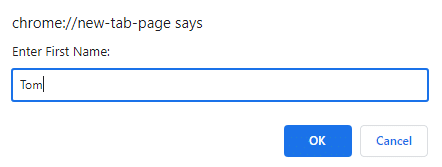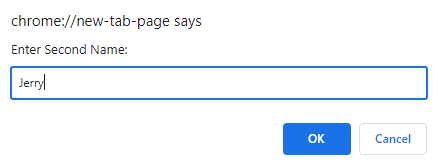## Conclusion:

As we saw, it is very important to understand JavaScipt Dollar Sign.

It will help to create more interactive websites by performing some complex arithmetic operations in the background of the HTML & CSS.

Though, the dollar sign in JavaScript is a very basic topic. But you should clear the concept to get a good grip on the advanced topic in the future.

Finally, thanks for reading, if the article was helpful to you feel free to comment and don’t forget to share.

Also if you are working towards becoming a better JavaScript developer  you can read javascript and jquery for front end web development.